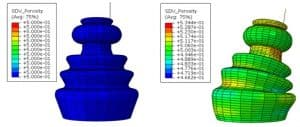rubber

## Modeling of porous tires with UHYPER subroutine

Subroutine: UHYPER
Language: Fortran
Solver: implicit/standard
Software: Abaqus
Appendices: tutorial video + manual + tutorial examplesPorous elastomeric materials are widely used in industry and have various applications such as shock absorbers, dampers, materials used in product packaging, and… Often in these types of uses, the material undergoes many deformations. In terms of product design and performance, engineers are interested in understanding the macroscopic response of these materials and their interaction with other components in the assembly set. Hence, the correct modeling of these materials under large deformations is one of the main design requirements in engineering applications.

In this program, a simple and correct model of the macroscopic elastic response of porous elastomers is assumed to be homogeneous. The model is implemented in the UHYPER sub-program of Abaqus software and is easy to use.

The UHYPER subroutine is intended to define the behavior of hyperelastic materials in Abaqus finite element software. This subroutine can be used in standard / implicit software solver.

# Metals

Subroutine: VUMAT
Language: Fortran
Solver: Explicit
Software: Abaqus
Appendices: instructional video + manual + instructional items
Version: 01.00

## What is GTNDamage?

This program is now ready for selective use in the industry to upgrade the GTN damage model available in Abaqus software. You can get the specifications of this software by contacting us.

GTN damage model:
The Gorson-Turgard-Needelman damage model, known as the GTN damage model, is one of the most well-known ductile failure criteria. The root of this criterion is micromechanical, and it is assumed that there are spherical holes in the space of the main material called the matrix. In this model, it is assumed that the material will have a ductile fracture when the plastic deformation in the metal causes the cavities to germinate, grow, and unite. The logic is that increasing the volume of cavities, called the volume percentage of cavities, reduces the stiffness of the material, and ultimately reduces the stiffness to such an extent that the material reaches the point of failure.

## GTN Damage Benchmark Features:

Theoretically, the GTN model has good flexibility to adapt to various mechanical behaviors such as plastic anisotropy, isotropic and kinematic strain, as well as viscoplasticity. Another is that it is possible to assume elliptical cavities in addition to spherical in this model and it can be developed.
In terms of computation and numerical modeling, the model developed in various finite element codes (FEM) is heavy and needs to be worked on more. In general, the implementation of a formulation in a computational code called Numerical Implementation is divided into two main parts in finite element books:
1- Numerical integration method
2- Tangent modulus calculation.
The choice of integration method and its adaptation to the tangential modulus is very important in the accuracy and stability of the current and the convergence of the finite element procedure.
In general, as you have probably seen and heard a lot, the method of numerical integration is divided into explicit (explicit) and implicit (implicit). In short, the explicit method is a simple but necessary method to solve the problem in small steps so as not to suffer from numerical instability. The implicit method is more complex than the explicit method, but it has much better accuracy and a significant convergence rate. The second part of implementing the formulation in finite element code is related to calculating the tangent modulus.
So far, 3 strategies have been used to calculate it. The first strategy is known as the Perturbation method, which is a numerical method. This method is simple but requires powerful CPUs for computing. Continuous tangential modulus is the second strategy used to calculate the tangential modulus.
This method is also simple, but because it has to calculate in small steps, it is slow to converge. The last strategy is the constrained tangential modulus. This method, unlike the previous two methods, is complex but has very good convergence.